# Test Prep Plan - Take a practice test

Take this practice test to check your existing knowledge of the course material. We'll review your answers and create a Test Prep Plan for you based on your results.
How Test Prep Plans work
1
2Based on your results, we'll create a customized Test Prep Plan just for you!
3Study smarter
Study more effectively: skip concepts you already know and focus on what you still need to learn.

# Applications of Integrals Chapter Exam

Exam Instructions:

Choose your answers to the questions and click 'Next' to see the next set of questions. You can skip questions if you would like and come back to them later with the yellow "Go To First Skipped Question" button. When you have completed the practice exam, a green submit button will appear. Click it to see your results. Good luck!

### Page 1

#### Question 1 1. What is the curve length of f(x) between x=0 and x=1?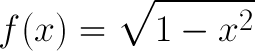#### Question 2 2. What is the equation for the volume of this cone that extends from x=0 to x=A, given that h=x?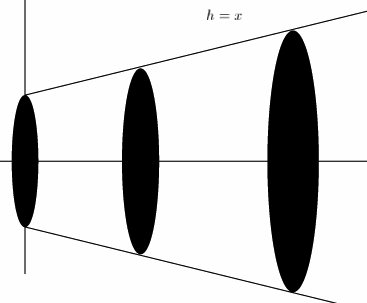#### Question 3 3. Consider the region bound by the x-axis, the y-axis, and f(x) between 0 and 4. What is an equation for the area of this region?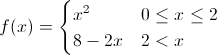#### Question 4 4. What is the area between f(x) and the x-axis from x=0 to x=2?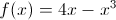#### Question 5 5. Given a velocity of v(t) as defined below, what is the equation for your position as a function of time?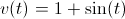### Page 2

#### Question 6 6. What is the volume of the cut-off cone shown?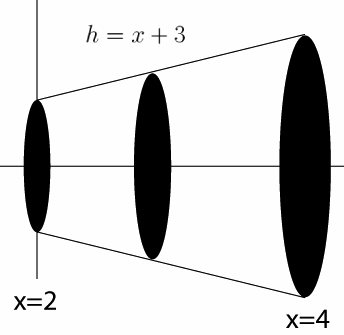#### Question 8 8. What is the equation for the area between f(x) and the x-axis from x=0 to x=2?### Page 3

#### Question 12 12. What is the equation for the volume of this cone that extends from x=0 to x=A, given that h=x?#### Question 13 13. Use integration to find the area enclosed by f(x) and g(x).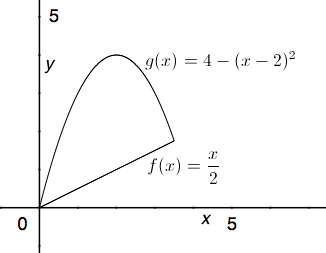#### Question 14 14. What is the equation for the volume of this cone that extends from x=0 to x=A, given that h=x?#### Question 15 15. Given the cut-off cone pictured, with h = x+3 and the region extending from x=2 to x=4, what is the integral you will need to solve to find the volume of this cut-off cone?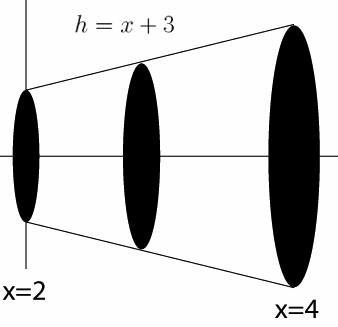### Page 4

#### Question 16 16. Use integration to find the area between the curves g(x) and f(x) between 0 and 1.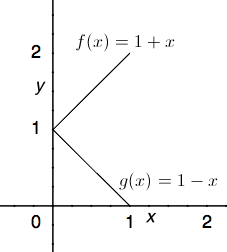#### Question 17 17. What is the volume made by revolving the area between g(x) and f(x) around the x-axis between x=0 and x=1?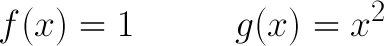#### Question 19 19. What is the volume made by revolving f(x) around the x-axis between x=0 and x=1?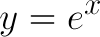#### Question 20 20. Given a velocity v(t) as defined below and a starting position of x=1 at t=0, what is the equation for your position as a function of time?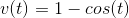### Page 5

#### Question 21 21. What is the equation for the length of the curve following y=f(x) between x=a and x=b given f(x) below?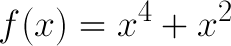#### Question 23 23. Use integration to find the area bounded by f(x), g(x), and h(x).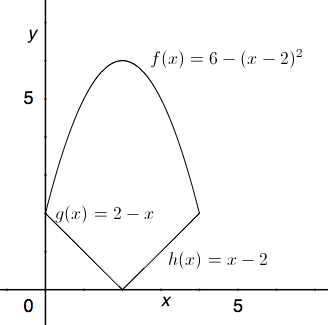#### Question 24 24. What is the length of f(x) between x=8 and x=15 for f(x) below?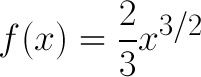#### Question 25 25. What is the volume made by revolving f(x) around the x-axis between x=0 and x=1?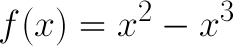### Page 6

#### Question 26 26. Use integration to find the area between the curves f(x) and g(x) between 0 and 4.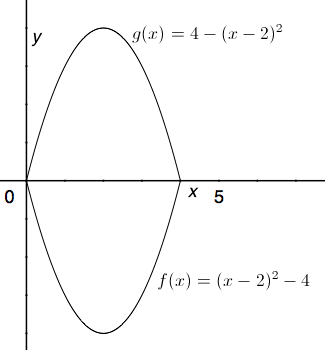#### Question 27 27. Consider the region bound by the x-axis, the y-axis, and f(x) between 0 and 4. What is the area of this region?#### Question 28 28. What is the area between f(x) and the x-axis from x=-2 to x=0?#### Question 29 29. Use integration to find the area between the curves h(x) and g(x) between 0 and 2.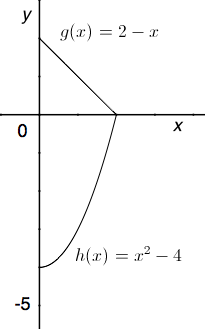#### Question 30 30. If your velocity is given by v(t), and you start at x=4 at t=0, then for what value of t will you be at the position x=20?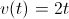#### Applications of Integrals Chapter Exam Instructions

Choose your answers to the questions and click 'Next' to see the next set of questions. You can skip questions if you would like and come back to them later with the yellow "Go To First Skipped Question" button. When you have completed the practice exam, a green submit button will appear. Click it to see your results. Good luck!

Support